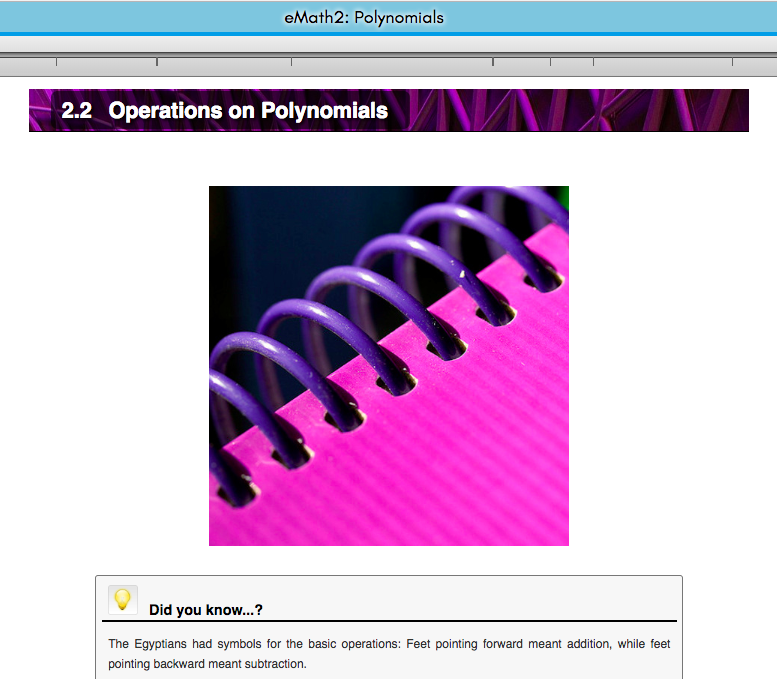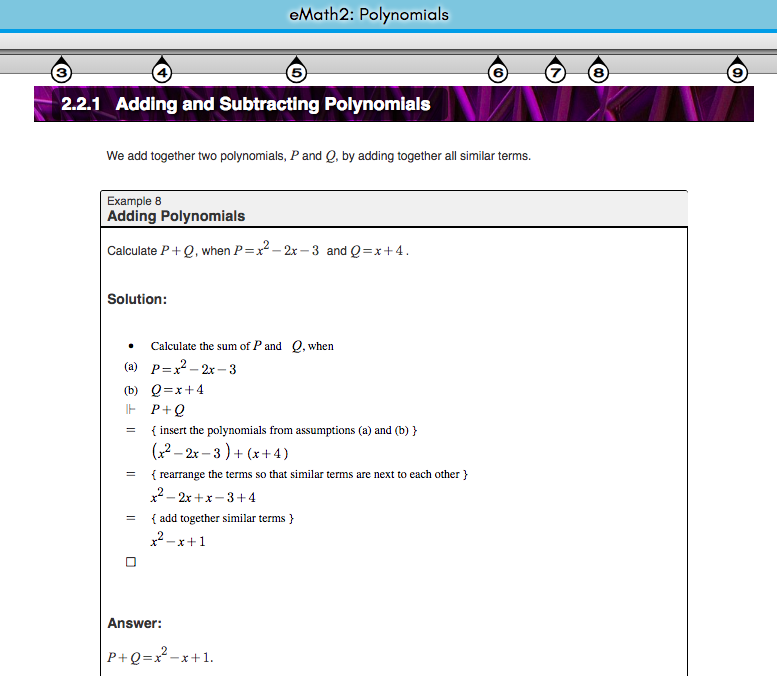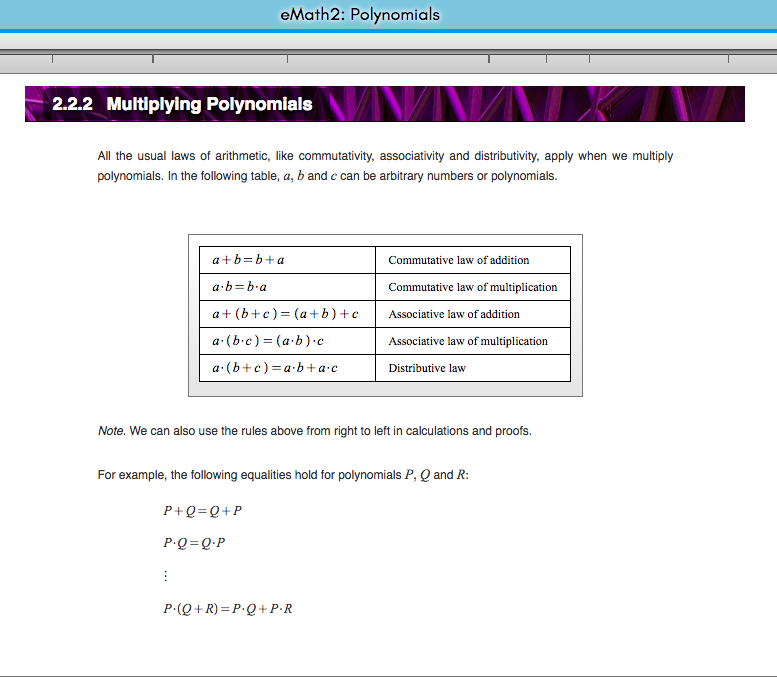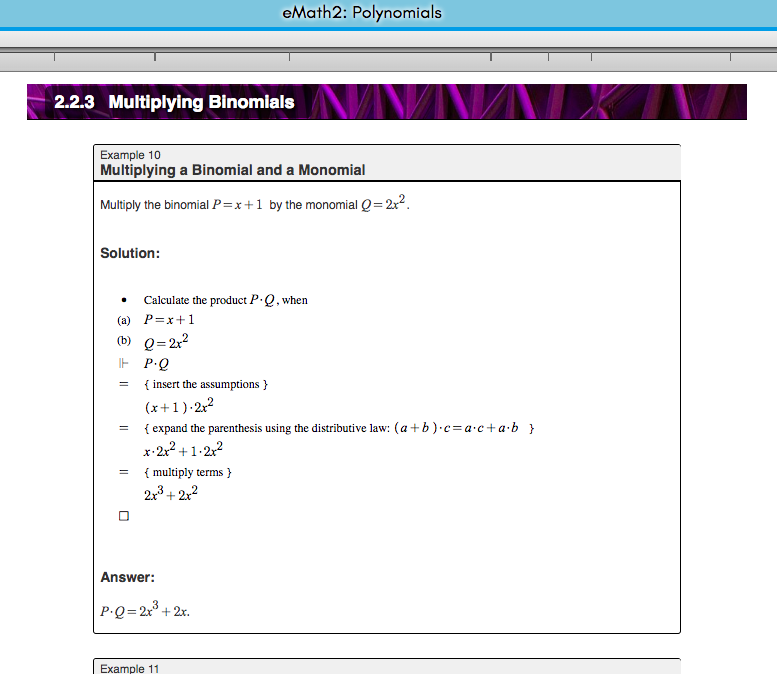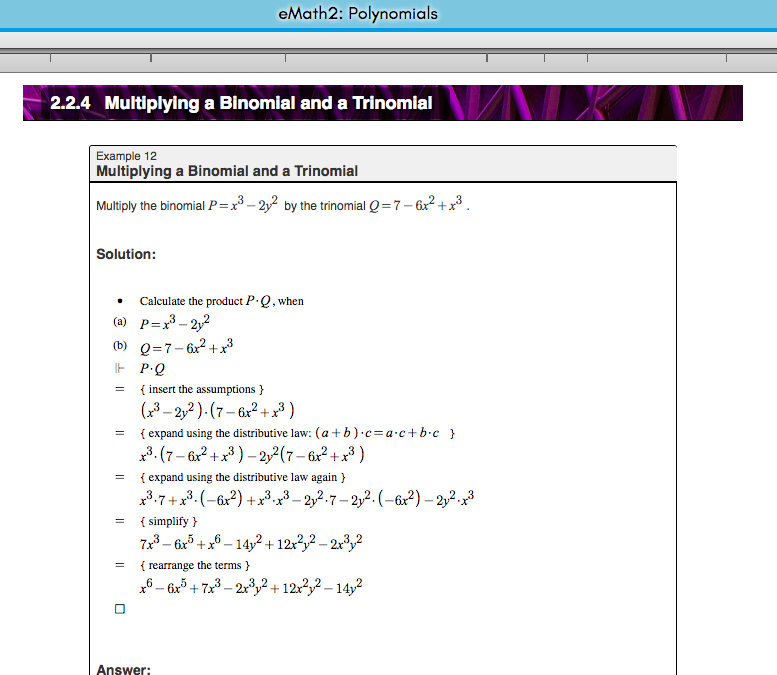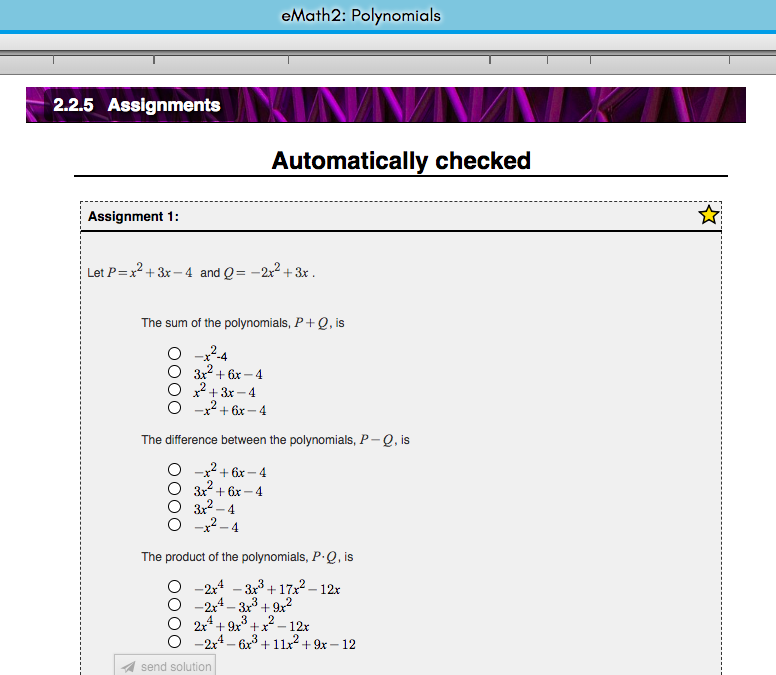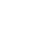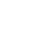# Self Study eMath Course

### Start learning today!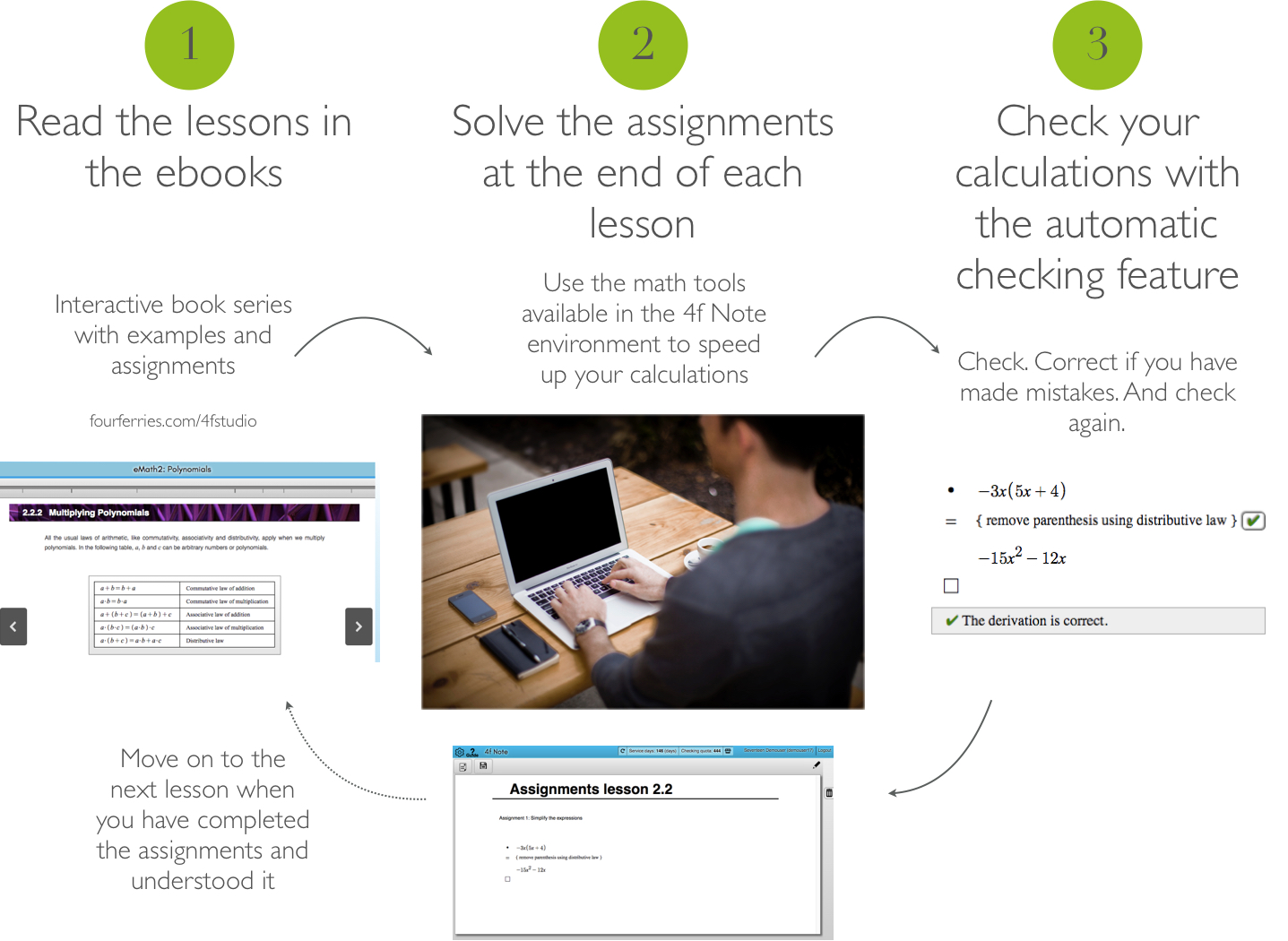eMath Self Study Course for Learning High School Mathematics

The ebook course is structured so that you will learn all concepts in a logical order and is meant to be studied from beginning to end. The theory parts are short and concise and at the end of each lesson, you will find a page with assignments.

All 10 course books in the eMath series in 4f Studio online, use as many or as few as you wish

4f Studio

Create your own electronic notebooks. Collect material, assignments, theory, all in one place.

4f Note

Effective tools for writing math digitally
Save pages where you have collected your own calculations, notes, material and links

The automatic checker

Check your calculations step-by-stepeMath1: Functions and equations

eMath1 is a repetition of middle school math, with some topics being treated more carefully than before. It gives an overview of the basic number domains and their operation, shows how solve first degree equations, how to work with percentages, roots and powers, and finally explains the important notion of a function.

eMath2: Polynomials

eMath2 presents first degree and higher degree polynomials. It shows how to solve equations involving polynomials, and how to factories polynomials. It also explains how to solve simultaneous equations as well as inequalities, and how to work with rational expressions.

eMath3: Geometry

eMath3 gives an overview of the basic concepts of plane geometry, and describes the theory of triangles, general polygons, and circles. It continues with an overview of solid geometry, with applications to cylinders, cones and spheres.

eMath4: Analytic Geometry

eMath4 introduces the basic notions of analytic geometry with the cartesian coordinate system. It shows how to describe lines as equations, and how to determine properties of lines, like intersection, orthogonality, distance from points and angles between lines. The book shows how to define and analyse properties of parabolas, circles and other conic sections.

eMath5: Vectors

eMath5 gives an overview of vectors and vector spaces. It explains the basic notion of a vector, how to compute with vectors and how to describe them in a coordinate system. Three-dimensional vectors are also described, together with planes and lines in space.

eMath6: Probability and Statistics

eMath6 gives an overview of the basic notions of probability and statistics. The basic statistical measures are covered, as well as surveys and samples. The concept of probability is explained, together with the basics of combinatorics. The rules of probability are explained, to show how to compute with probabilities, and the notion of a random variable is explained.

eMath7: Derivatives

eMath7 introduces the basic notions of calculus: continuity, limits, and derivatives. It shows how to compute the derivative of a function. It applies this to describe the behavior of functions, and to find extreme values of functions. Additionally, it includes a chapter on how to work with rational expressions.

eMath8: Logarithms and Roots

eMath8 describes how to work with exponents, logarithms and roots. It explains the notions of compound functions and inverse functions. It introduces exponential functions and logarithmic functions and shows how compute the derivative of these.

eMath9: Trigonometric Functions and Sequences

eMath9 gives a more in-depth treatment of trigonometry. It covers the basic trigonometric functions, how to compute with these and how to solve equations with trigonometric functions. The book also covers sequences in general, particularly arithmetic and geometric sequences, as well as arithmetic and geometric sums.

eMath10: Integral Calculus

eMath10 shows how to define integrals as the inverse of derivatives. It introduces the notion of a definite integral, and shows how to use this in area calculations. Definite integrals are then used to also calculate volumes of solids.### Subscribe to a self study course

Subscribing to the online course will give you

• Access to all books in the eMath series
• Access to 4f Note where you can do your assignments and check them with the checker
• 200 Checkings/ month subscribed

### Examples and assignments

The books are clearly structured with one new theoretical issue introduced per page. The ebooks include both examples and assignments with answers. Assignment pages are found at the end of each chapter. These can be used as lesson exit tickets to check that the student has mastered the theory introduced in the chapter.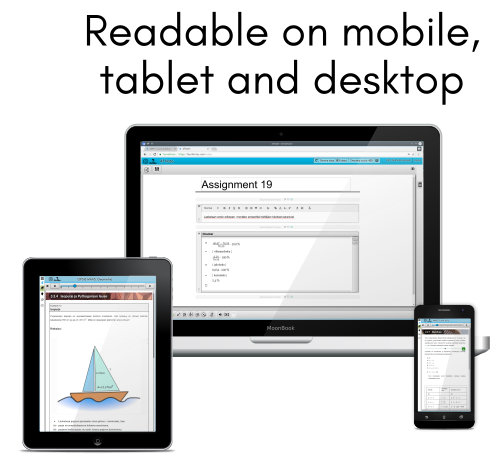### Automatic checking included

In addition course subscribers have access to the automatic checker that checks the calculations step-by-step.  You can use the checker to correct your work and spot where you have made mistakes, when working in the 4fNote environment.

### Effective tools for Math

4f Note provides a powerful collection of tools for digital mathematics education. The rich text editor uses traditional mathematical notation for formulas, so mathematical text can be written and edited in a simple and straightforward way.  You can add a number of different mathematical content elements to a page, like function graphs, geometric figures, displayed formulas, calculations in traditional format as well as in structured derivation format, sign charts and general mathematical tables. Learn more...

# Have a look inside the book

### Developed by Math teachers and researchers from Finland, Sweden and Estonia

The eMath textbooks used in the course are developed by Math teachers from Finland, Sweden and Estonia. The books follow a teaching approach that uses the Structured Derivations methodology, where the idea is that every step has an explicit justification. This makes it easy to follow the examples that explain the theory. At the same time the approach forces the student to reflect on what they are doing. The books also introduce the students to logic, a step often skipped in school, but without which mathematics becomes magic instead of reasonable and understandable.